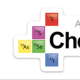# You can teach most of buffers without teaching buffers, if you likeJune 06, 2022

Routinely, buffers are cited by AP chemistry teachers as the topic that their students find the most challenging. Not necessarily equilibrium, but buffers. So, what about essentially largely avoiding the teaching of buffers completely, and approaching the problem from an equilibrium viewpoint instead? Let’s consider an example calculation.

20.0 mL of 0.100 M ethanoic acid (Ka = 1.80 x 10-5) is titrated with 6.00 mL of 0.100 M NaOH. Calculate the pH of the resulting solution.

Method 1, treat it like the buffer solution that it is by using the Henderson-Hasselbalch equation

To most teachers this looks like the partial neutralization of a weak acid with a strong base that yields a buffer solution in the Erlenmeyer flask. As soon as I see a buffer being made, my advice to the kids is, “use the Henderson-Hasselbalch equation”. Here’s the math that goes with that realization.

• Moles of acid initially = (20.0/1000)(0.100) =0.00200 mols
• Moles of NaOH initially = (6.00/1000)(0.100) = 0.000600 mols
• Ratio 1:1:1:1, (CH3COOH + OH → CH3COO + H2O), so NaOH is limiting
• Moles of acid after reaction = 0.00200 – 0.000600 = 0.00140 mols
• Moles of CH3COO produced = 0.000600 mols
• Total volume in the flask = (20.0 + 6.00)/1000 = 0.026 L
• Apply HH, and we get pH = -log (1.80 x 10-5) + log [(0.000600/0.026)/(0.00140/0.026)] = 4.38

However, what if the student simply doesn’t see it as a buffer?

Method 2, treat it essentially like a common ion/equilibrium problem, by using an ICE table and Ka.

Firstly, let’s consider the reaction of the acid and the base already described above that leaves 0.00140 moles of acid, and construct the ICE table for the dissociation of that remaining acid in water in red below. Now let’s treat this like a common ion problem, and add-in the moles of CH3COO created by the reaction that occurred on the addition of the NaOH in blue. If we consider X to be negligible then we get the green numbers.

 CH3COOH + H2O → CH3COO– + H3O+ 0.00140 0 0 – X + X + X 0.00140 – X 0 + X + 0.000600 0 + X 0.00140 0.000600 X

Now we can apply the original Ka value as if this were a common ion problem (recalling that the total volume of solution at this point is 26.0 mL), to getSolving for [H3O+] = 4.2 x 10-5

pH = -log(4.2 x 10-5) = 4.38, which is of course what we would expect if the methods are comparable.

1. It doesn’t really completely eliminate the need to teach buffers per se, because students still have to know what they are, and how they interact with acids and bases etc.

2. Many people will argue that these are absolutely NOT ‘alternative approaches’ to the calculation, since when you realize that the H-H equation is simply the Ka expression logged all the way through (do the math and see that the HH is derived from the Ka expression), you could argue that all you are ever doing is applying the second method.

Despite those potential criticisms, I still think this offers a potentially useful alternative thought process, and frankly, the more you know and understand the easier this whole thing becomes.

1.Hey Adrian – I stumbled upon another idea which may help too. Why not just use the Ka expression without the ICE table or the HH.? Of course the kids would have to recognize that it’s a buffer

Use [H3O+] = Ka(acid)
(base)

so.. [H3O] = 1.8×10-5 (Acid = start 0.002moles – 0.0006 base added = 0.0014)
(Base = start “0” moles + 0.0006 base = 0.0006)

solve and -log to the pH

I don’t know – I’m new at the AP thing but almost any buffer problem can be answered using this basic Eq equation and it seems easy to understand how/why things are happening.

•Well that’s fine, but that’s only a different version of HH, and one that doesn’t appear on the equations and constant packet so that presents a problem. What I’m saying is that you can solve this issue by reverting back to what some kids might think is a topic that they’ve already mastered.

•I noticed that this approach works for my students. I give them a practice problem on acids when I teach equilibrium. That way they have already seen it and panic doesn’t set in as much.